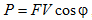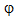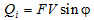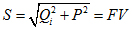ISSN (print) 1995-2732
ISSN (online) 2412-9003

Abstract

The types of mechanical power in vibration processes are under study. When vibration machines work, kinetic energy is generated due to the movement of massive bodies and thermal energy is produced due to friction. Their derivatives define different types of mechanical power: variable reactive and nonnegative thermal types. A dual-inverse analogue of reactive mechanical power is reactive electrical power. Thermal mechanical power corresponds to the electrical active power. The aim of this paper is to give a theoretical description of the varieties of power that occur during the operation of vibration machines. The task is an analytical representation of the energy aspect of vibration phenomena. The relevance of the study is due to the negative effect of mechanical reactive power on the quality of supply current (the appearance of harmonics with the frequency of mechanical vibrations, the transformation of mechanical reactive power into electrical reactive power, etc.). The study is based on the fact that in a satisfactory approximation the movement of a massive working body of the vibration mechanism is deemed to be harmonic, just as active power of electrical engineering is determined by the mechanical dissipative power, whereis the phase difference of oscillations of the presented values, just as reactive power of electrical engineering is determined by the mechanical reactive (inertial) power. In electrical engineering it is assumed that P is an average value, and Q is the amplitude. Everything is the same in this case, just as in electrical engineering total power is determined:. It is calculated as a multiplication of the effective values of the quantities. Complex and vector descriptions of dissipative and reactive power are presented. The tangible embodiment of virtual rotating vectors in vibration processes are cranks of rotational and linear transducers.

Keywords

Reactive power, active power, full power, complex representation, vector representation.

Igor P. Popov – Assistant Professor

Kurgan State University. E-mail: This email address is being protected from spambots. You need JavaScript enabled to view it.